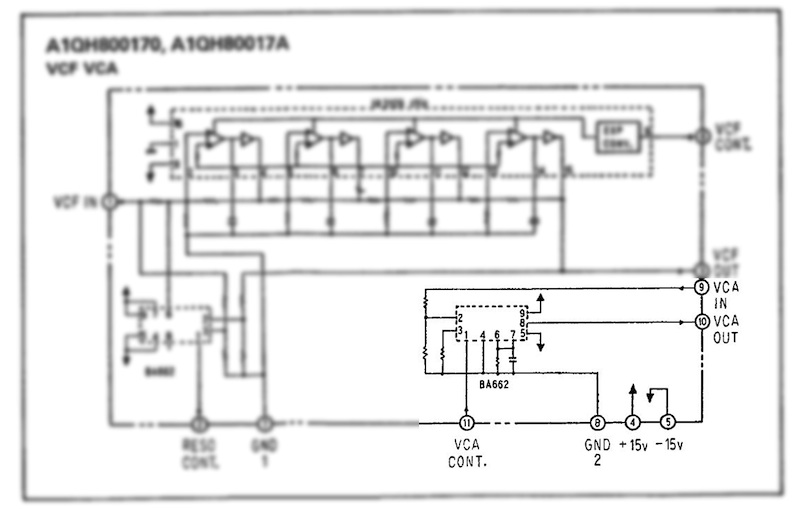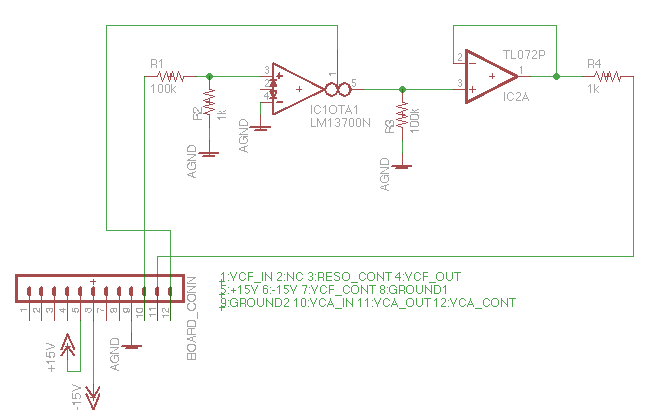# VCA part done (well almost)

80017a VCA open source hardware replacement is done for about 95%.

Original Roland:See previous post for Mooger5’s working BA6110 IC solution. Since I’m going to use the LM13700 OTA for the filter part, I figured the VCA would be a nice exercise in OTA building. To fully understand the inner workings of an OTA, I followed great lectures by Aaron Lanterman on Electronics for Musical Synthesis!

My VCA version:

First some osc measurements.

Pin 3 VCF OUT has a voltage level between 2.4V and -2.4V (as stated in the tuning instruction, TP19 for voice 1, 4.8Vpp)

Pin 9 VCA IN has a voltage level between 0V and -1.4V

Pin 11 VCA CONT has an unknown current because my multimeter is “current broken”. Various datasheet and internet resources mention a current < 5mA. OK for the moment.

Pin 10 VCA OUT

The schematic (power and unused IC parts not shown!)Pin numbers are different because in this header configuration, pin2 is not connected (like on the Juno board)

VCA IN delivers a voltage between 0V and -1.4V. The OTA requires an input V p.p. of around 10mV. The VCA IN voltage has to be divided by 100, to |0.014V|, |14mV|. R1 and R2 make this division.

Maximum Amp Bias Input (OTA pin 1, Juno VCA CONT) is 0.5mA (assumption, not measured). This makes the maximum gain of the OTA 19.2 (gm) * 0.5mA ≈ 20 * 0.5mA = 10 m℧ (10 millimho’s). The OTA’s max Iout is Vdiff * 10m℧ = 14mV * 10m℧ = 140*10^-6 = 0.14mA.

The current after OTA pin 5 at max gain is, as calculated, 0.14mA. To convert this current back to a voltage we’ll use a resistor to ground. The value of this resistor is: V=I*R, the wanted V at maximum gain equals the V at the OTA input. R3 = target_voltage / I = 1.4V/0.14mA = 100kΩ.

The TL072 serves as output buffer. The 1k resistor protects the preceding circuit. Result goes to pin 11, VCA OUT

So, why almost done? This VCA has just a little less gain than the original VCA’s. The max amp bias input is probably not exacly 0.5mA at max. When I have a new multimeter (or borrowed one), I’ll measure the current and redo my calculations.